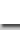Research Fellow/Professor  |  Wang, Bow-Yaw

 Research Descriptions Compositional reasoning is one of the most effective checking. In compositional reasoning, the verification of a system is decomposed into simpler verification tasks of its components according to certain proof rules. By the soundness of compositional proof rules, the correctness of the system follows from the correctness of its components. Since components are verified separately, the state explosion problem is therefore circumvented. Developing sound compositional proof rules however depends on properties and computational models. Indeed, significant research effort has been devoted to it for decades. For finite-state automata and trace semantics, we have developed an automated technique to derive compositional proof rules. Moreover, we have shown that the class of prefix-closed regular languages forms a Heyting algebra. The proof system LJ for intuitionistic logic is thus able to derive compositional proof rules for the subclass of regular languages.        Model checking and theorem proving are two of the most promising techniques in formal verification. In model checking, a mathematical model of computer system is checked against its formal requirements. The model-theoretic technique is able to explore finite-state models rather efficiently and widely applied in the design of computer hardware systems. On the other hand, computer systems are formalized as logical theories in theorem proving. Verification of systems amounts to deducing mathematical theorems from these logical theories. The proof-theoretic technique is able to check mathematical proofs formally. Due to the expressiveness of theorem proving, it is generally used in infinite-state systems such as computer software systems. Either technique has its strength and weakness. Neither can verify arbitrary computer systems satisfactorily. One of the most exciting research problems is therefore to develop a hybrid technique which combines advantages of both techniques.        My research interests include both model checking and theorem proving techniques in formal verification. In model checking, we developed a complete SAT-based model checking algorithm based on a proof-theoretic framework. The model checking algorithm is reduced to proof search in the framework. Our SAT-based algorithm uses modern SAT solvers to search proofs and hence solve the model checking problem. Our proof-theoretic approach is in fact more general than typical SAT-based model checking. Firstly, the new approach is able to verify properties in the universal fragment of mu-calculus which is more expressive than the universal fragment of CTL* and hence linear temporal logic. Secondly, it has been applied to the verification of context-free processes almost straightforwardly. Our approach can not only verify more properties but also infinite-state systems. Traditional SAT-based technique has yet to be generalized in both accounts.        The very first step in applying theorem proving is to formalize problems in the chosen prover. In formal verification, one has to embed various temporal logics and computational models in a prover and carry out proofs formally. Indeed, both linear temporal logic and computation tree logic has been embedded in various theorem provers. However, the formalization task needs to be carried out again should different temporal logic or computational model be used in specific application. We develop a modular formalization of CTL* to address the problem. CTL* is strictly more expressive than both linear temporal logic and computation tree logic. Moreover, our modular formalization states computation assumptions explicitly in the Coq module system. To reuse our formalization, one only needs to establish these assumptions formally. Using our modular formalization, we have established most of the soundness theorems for LTL, UB, CTL, and CTL*. Due to our modular formalization, these theorems need not be re-established when they are instantiated in future applications.